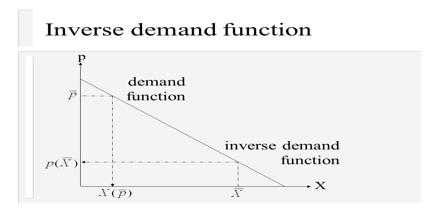Economics

# Inverse Demand FunctionInverse Demand Function is not the reciprocal of the demand function—the word “inverse” refers to the mathematical concept of an inverse function. It is a function p(q) that maps from a quantity of output to a price in the market; one might model the demand a firm faces by positing an inverse demand function and imagining that the firm chooses a quantity of output. It can be used to derive the total and marginal revenue functions. It is the form of the demand function that appears in the famous Marshallian Scissors diagram.